• Fisher算法python实现含训练集和测试集，Fisher算法也叫Fisher判别分析，或线性判别分析（LDA）
• 上因为最早由[Fisher, 1936]提出,亦称“Fisher判别分析”。 LDA的基本思想:给定训练样例集,设法将样例投影到一条直线上,使得同类样例的投影点尽可能接近、异类样例的投影点尽可能远离;在对新样本进行分类时,将其投影...
• 文章目录一、线性分类的数学基础与应用1、Fisher基本介绍2、Fisher判别思想3、举例二、Fisher判别的推导（python）1、代码2、代码结果三、Fisher分类器1、定义2、scikit-learn中LDA的函数的代码测试3、监督降维技术...
• 二、Fisher判别分析的思想三、Fisher判别分析步骤四、Fisher判别分析python代码实现 一、什么是Fisher线性判别？ 可以考虑把d维空间的样本投影到一条直线上，形成一维空间，即把维数压缩到一维，这在数学上容易办到...

一、什么是Fisher线性判别？

可以考虑把d维空间的样本投影到一条直线上，形成一维空间，即把维数压缩到一维，这在数学上容易办到，然而，即使样本在d维空间里形成若干紧凑的互相分得开的集群，如果把它们投影到一条任意的直线上，也可能使得几类样本混在一起而变得无法识别。但是在一般情况下，总可以找到某个方向，使得在这个方向的直线上，样本的投影能分开得最好。问题是如何根据实际情况找到这条最好的、最易于分类的投影线，这就是Fisher算法所要解决的基本问题。

二、Fisher判别分析的思想

Fisher判别的思想是投影，将R组P维数据投影到某一个方向，用一元方差分析的方法使投影组与组之间尽可能分开。
如下所示，给出一个二维示意图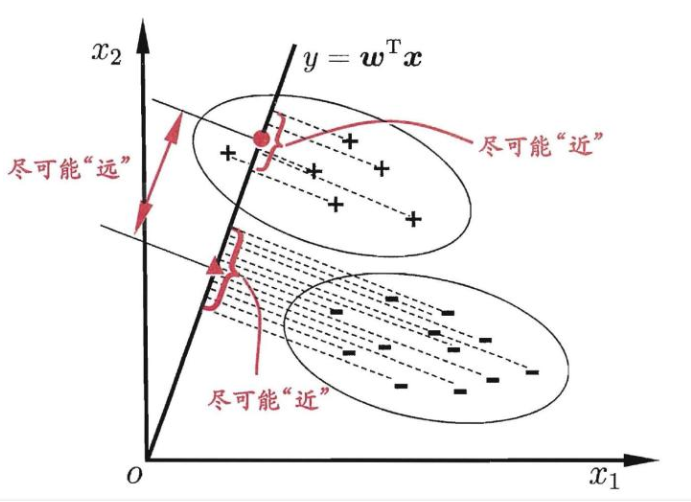上 面 二 维 示 意 图 中 的 " + " 、 " − " 分 别 代 表 正 例 和 反 例 ， 椭 圆 表 示 数 据 簇 的 外 轮 廓 ， 虚 线 表 示 投 影 ， 红 色 实 心 圆 和 红 色 实 心 三 角 形 分 别 表 示 两 类 样 本 投 影 后 的 中 心 点 。 上面二维示意图中的"+"、"-"分别代表正例和反例，椭圆表示数据簇的外轮廓，虚线表示投影，红色实心圆和红色实心三角形分别表示两类样本投影后的中心点。 给 定 数 据 集 , D ϵ { ( X i , Y i ) } ， , Y i ϵ ( 0 , 1 ) 令 X i 、 μ i 分 别 表 示 第 , i ϵ { 0 , 1 } 类 示 例 的 集 合 、 均 值 向 量 。 给定数据集 ,D\epsilon \{(X_i,Y_i)\}，,Y_i\epsilon {(0,1)}令 X_i、 \mu_i 分别表示第,i\epsilon\{0,1\} 类示例的集合、均值向量。 若 将 数 据 投 影 到 直 线 ω 上 ， 则 两 类 样 本 的 中 心 在 直 线 上 的 投 影 分 别 为 ω T μ 0 和 ω T μ 1 。 若将数据投影到直线 \omega上，则两类样本的中心在直线上的投影分别为\omega^T\mu_0和\omega^T\mu_1 。

三、Fisher判别分析步骤

1. 把 来 自 2 类 的 训 练 样 本 集 划 分 为 2 个 子 集 x 0 和 x 1 ； 1. 把来自2类的训练样本集划分为2个子集 x_0 和 x_1 ；

2. 计 算 各 类 的 均 值 向 量 μ i , i = 0 , 1 ； μ i = 1 N i ∑ x ϵ X i x ； 2. 计算各类的均值向量 \mu_i , i = 0,1； \mu_i =\frac{1}{N_i}\sum_{x\epsilon X_i }x；

3. 计 算 各 类 的 类 内 离 散 矩 阵 S ω i ， i = 0 , 1 ； S ω i = ∑ x ϵ X i ( x − μ i ) ( x − μ i ) T ； 3. 计算各类的类内离散矩阵 S_{\omega_i}， i = 0,1 ；S_{\omega_i} = \sum_{x\epsilon X_i }(x - \mu_i)(x - \mu_i) ^T；

4. 计 算 类 内 总 离 散 矩 阵 S ω = S ω 0 + S ω 1 ； 4. 计算类内总离散矩阵 S_\omega = S_{\omega_0} + S_{\omega_1}；

5. 计 算 矩 阵 S ω 的 逆 矩 阵 S ω − 1 ； 5. 计算矩阵 S_\omega 的逆矩阵S_\omega^{-1}；

6. 求 出 向 量 ω ∗ = S ω − 1 ( μ i − μ 0 ) （ 此 处 采 用 拉 格 朗 日 乘 子 法 解 出 F i s h e r 线 性 判 别 的 最 佳 投 影 方 向 ） ； 6. 求出向量\omega^* = S_\omega^{-1}(\mu_i - \mu_0)（此处采用拉格朗日乘子法解出Fisher线性判别的最佳投影方向）；

7. 判 别 函 数 为 y = ω ∗ T x ； 7. 判别函数为 y = {\omega^*}^Tx；

8. 判 别 函 数 的 阈 值 ω 0 可 采 用 以 下 两 种 方 法 确 定 ： 8. 判别函数的阈值{\omega_0}可采用以下两种方法确定： 第 一 种 是 ω 0 = ω ∗ T μ 0 + ω ∗ T μ 1 2 ； 第一种是 {\omega_0} = \frac{{\omega^*}^T\mu_0 + {\omega^*}^T\mu_1}{2}；
第 二 种 是 ω 0 = N 0 ω ∗ T μ 0 + N 1 ω ∗ T μ 1 N 0 + N 1 ； 第二种是 {\omega_0} = \frac{N_0{\omega^*}^T\mu_0 + N_1{\omega^*}^T\mu_1}{N_0 + N_1} ；

9. 分 类 规 则 ： 比 较 y 值 与 阈 值 ω 0 的 大 小 ， 得 出 其 分 类 。 9. 分类规则：比较 y 值与阈值{\omega_0} 的大小，得出其分类。

四、Fisher判别分析python代码实现

导入包

#导入包
import pandas as pd
import numpy as np
import matplotlib.pyplot as plt
import seaborn as sns

导入数据集

#导入数据集
Iris1=df.values[0:50,0:4]
Iris2=df.values[50:100,0:4]
Iris3=df.values[100:150,0:4]

定义类均值向量

#定义类均值向量
m1=np.mean(Iris1,axis=0)
m2=np.mean(Iris2,axis=0)
m3=np.mean(Iris3,axis=0)

定义类内离散度矩阵

#定义类内离散度矩阵
s1=np.zeros((4,4))
s2=np.zeros((4,4))
s3=np.zeros((4,4))
for i in range(0,30,1):
a=Iris1[i,:]-m1
a=np.array([a])
b=a.T
s1=s1+np.dot(b,a)
for i in range(0,30,1):
c=Iris2[i,:]-m2
c=np.array([c])
d=c.T
s2=s2+np.dot(d,c)
for i in range(0,30,1):
a=Iris3[i,:]-m3
a=np.array([a])
b=a.T
s3=s3+np.dot(b,a)

定义总类内离散矩阵

#定义总类内离散矩阵
sw12 = s1 + s2;
sw13 = s1 + s3;
sw23 = s2 + s3;

定义投影方向

#定义投影方向
a=np.array([m1-m2])
sw12=np.array(sw12,dtype='float')
sw13=np.array(sw13,dtype='float')
sw23=np.array(sw23,dtype='float')

判别函数以及阈值T（即w0）

#判别函数以及阈值T（即w0）
a=m1-m2
a=np.array([a])
a=a.T
b=m1-m3
b=np.array([b])
b=b.T
c=m2-m3
c=np.array([c])
c=c.T
w12=(np.dot(np.linalg.inv(sw12),a)).T
w13=(np.dot(np.linalg.inv(sw13),b)).T
w23=(np.dot(np.linalg.inv(sw23),c)).T
T12=-0.5*(np.dot(np.dot((m1+m2),np.linalg.inv(sw12)),a))
T13=-0.5*(np.dot(np.dot((m1+m3),np.linalg.inv(sw13)),b))
T23=-0.5*(np.dot(np.dot((m2+m3),np.linalg.inv(sw23)),c))

计算正确率

#计算正确率
kind1=0
kind2=0
kind3=0
newiris1=[]
newiris2=[]
newiris3=[]
for i in range(30,49):
x=Iris1[i,:]
x=np.array([x])
g12=np.dot(w12,x.T)+T12
g13=np.dot(w13,x.T)+T13
g23=np.dot(w23,x.T)+T23
if g12>0 and g13>0:
newiris1.extend(x)
kind1=kind1+1
elif g12<0 and g23>0:
newiris2.extend(x)
elif g13<0 and g23<0 :
newiris3.extend(x)
for i in range(30,49):
x=Iris2[i,:]
x=np.array([x])
g12=np.dot(w12,x.T)+T12
g13=np.dot(w13,x.T)+T13
g23=np.dot(w23,x.T)+T23
if g12>0 and g13>0:
newiris1.extend(x)
elif g12<0 and g23>0:
newiris2.extend(x)
kind2=kind2+1
elif g13<0 and g23<0 :
newiris3.extend(x)
for i in range(30,49):
x=Iris3[i,:]
x=np.array([x])
g12=np.dot(w12,x.T)+T12
g13=np.dot(w13,x.T)+T13
g23=np.dot(w23,x.T)+T23
if g12>0 and g13>0:
newiris1.extend(x)
elif g12<0 and g23>0:
newiris2.extend(x)
elif g13<0 and g23<0 :
newiris3.extend(x)
kind3=kind3+1
correct=(kind1+kind2+kind3)/60

判断出来的综合正确率

print('正确率：',correct*100,'%')

运算结果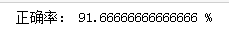最终判断出来的综合正确率为91.66666666666666%！

展开全文• fisher判别分析原理+python实现

万次阅读 多人点赞 2017-05-03 20:07:41
参考资料： 周志华老师的《机器学习》 ...判别分析是一种经典的现行分析方法，其利用...在这里我们主要讨论fisher判别分析的方法。fishter原理费歇（FISHER判别思想是投影，使多维问题简化为一维问题来处理。选择一个

参考资料：

判别分析是一种经典的现行分析方法，其利用已知类别的样本建立判别模型，对未知类别的样本进行分类。在这里我们主要讨论fisher判别分析的方法。

fishter原理

费歇（FISHER）判别思想是投影，使多维问题简化为一维问题来处理。选择一个适当的投影轴,使所有的样品点都投影到这个轴上得到一个投影值。对这个投影轴的方向的要求是：使每一类内的投影值所形成的类内离差尽可能小，而不同类间的投影值所形成的类间离差尽可能大。

公式推导

这里给出一个二维的示意图（摘自周志华老师的《机器学习》一书），在接下来的讨论中我们也将以二维的情况做分类来逐步分析原理和实现。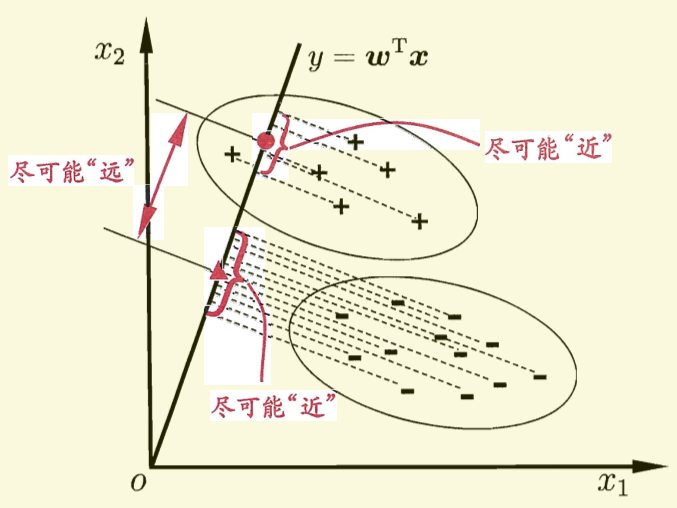ps: 图中有一处描述似乎不是特别的准确，直线的方程应该是

0=wTx

而不是
y=wTx

ps: 因为在书关于此的其他讨论中，并未涉及任何y的概念，这里将y写入对我造成了某种误导。

对于给定的数据集，D（已经设置好分类标签）， Xi,Ui,i $X_i, U_i, \sum_i$分别表示给定类别 i $i$ 的集合，均值向量，协方差矩阵。现将数据投影到直线x=0$x = 0$ 上，则样本中心的投影为 0=w1u1+w2u2++wnun $0 = w_1*u_1 + w_2 * u_2 + \dots +w_n * u_n$。(n 为样本维度，接下来的讨论中将统一设置为2)，写成向量形式则为 wTu=0 $w^T u= 0$ 如果将所有的样本都投影到直线上，则两类样本的协方差分别为 wT0wwT1w $w^T\sum_0w 和 w^T\sum_1w$。要想达到较好的分类效果，应该是的同类样本的投影点尽可能的接近，也就是让同类样本投影点的协方差尽可能的小。即 (wT0w+wT0w) $(w^T\sum_0w + w^T\sum_0w)$ 尽可能小。同时也应该保证不同类样本投影点尽可能的互相远离，即 wTu0wTu1 $\left \| w^Tu_0 - w^Tu_1 \right \|$ 尽可能大。如果同时考虑两者的关系可以得到下面需要最大化的目标：

J=wTu0wTu1wT0w+wT0w

这里定义“类内散度矩阵”（within-class scatter matrix）
Sw=0+1=xX0(xu0)(xu0)T+xX1(xu1)(xu1)T

以及类间离散度矩阵（between-class scatter matrix）
Sb=(u0u1)(u0u1)T

J $J$可重写为：
J=wTSbwwTSww

ps:sorry 这些公式确实敲得有点累，道个歉，我直接截图了。希望不影响大家的理解。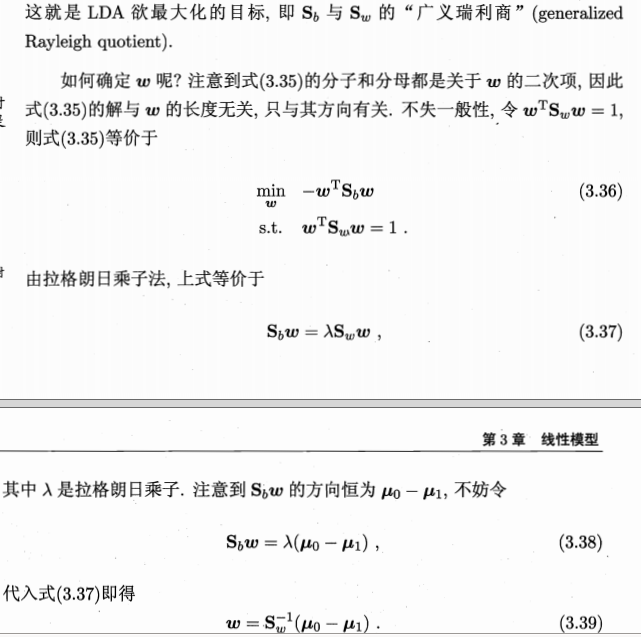在推导出上面的公式之后我们就可以开始写代码了。

编程实现

数据生成

这里我偷一个懒，直接用scikit-learn的接口来生成数据：

from sklearn.datasets import make_multilabel_classification
import numpy as np

x, y = make_multilabel_classification(n_samples=20, n_features=2,
n_labels=1, n_classes=1,
random_state=2)  # 设置随机数种子，保证每次产生相同的数据。

# 根据类别分个类
index1 = np.array([index for (index, value) in enumerate(y) if value == 0])  # 获取类别1的indexs
index2 = np.array([index for (index, value) in enumerate(y) if value == 1])  # 获取类别2的indexs

c_1 = x[index1]   # 类别1的所有数据(x1, x2) in X_1
c_2 = x[index2]  # 类别2的所有数据(x1, x2) in X_2

fisher算法实现

def cal_cov_and_avg(samples):
"""
给定一个类别的数据，计算协方差矩阵和平均向量
:param samples:
:return:
"""
u1 = np.mean(samples, axis=0)
cov_m = np.zeros((samples.shape, samples.shape))
for s in samples:
t = s - u1
cov_m += t * t.reshape(2, 1)
return cov_m, u1

def fisher(c_1, c_2):
"""
fisher算法实现(请参考上面推导出来的公式，那个才是精华部分)
:param c_1:
:param c_2:
:return:
"""
cov_1, u1 = cal_cov_and_avg(c_1)
cov_2, u2 = cal_cov_and_avg(c_2)
s_w = cov_1 + cov_2
u, s, v = np.linalg.svd(s_w)  # 奇异值分解
s_w_inv = np.dot(np.dot(v.T, np.linalg.inv(np.diag(s))), u.T)
return np.dot(s_w_inv, u1 - u2)

判定类别

def judge(sample, w, c_1, c_2):
"""
true 属于1
false 属于2
:param sample:
:param w:
:param center_1:
:param center_2:
:return:
"""
u1 = np.mean(c_1, axis=0)
u2 = np.mean(c_2, axis=0)
center_1 = np.dot(w.T, u1)
center_2 = np.dot(w.T, u2)
pos = np.dot(w.T, sample)
return abs(pos - center_1) < abs(pos - center_2)

w = fisher(c_1, c_2)  # 调用函数，得到参数w
out = judge(c_1, w, c_1, c_2)   # 判断所属的类别
print(out)

绘图

import matplotlib.pyplot as plt

plt.scatter(c_1[:, 0], c_1[:, 1], c='#99CC99')
plt.scatter(c_2[:, 0], c_2[:, 1], c='#FFCC00')
line_x = np.arange(min(np.min(c_1[:, 0]), np.min(c_2[:, 0])),
max(np.max(c_1[:, 0]), np.max(c_2[:, 0])),
step=1)

line_y = - (w * line_x) / w
plt.plot(line_x, line_y)
plt.show()

最后一步【贴图】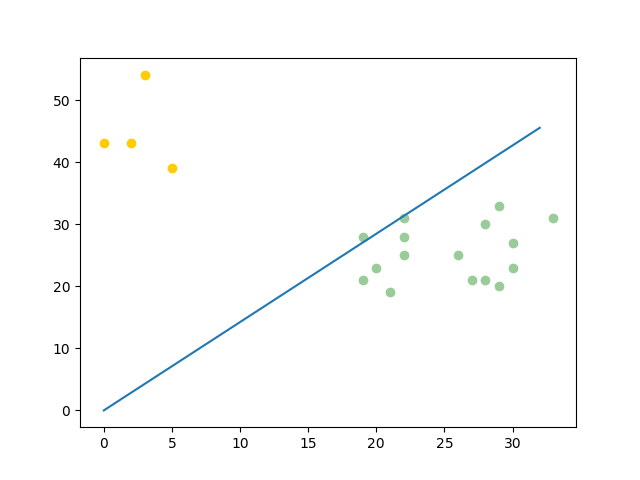最后的最后，大家只要把上面所有的代码复制粘贴到一个文件夹下，在python3 环境下运行就好了。本人调试运行的环境为：

• python3
• ubuntu 16.04
• pycharm
展开全文python fisher 机器学习 线性判别分析
• python代码完成Fisher判别

千次阅读 2020-05-05 22:44:24
文章目录一．算法描述（1）W的确定（2）阈值的确定（3）...Fisher线性判别分析的基本思想：选择一个投影方向（线性变换，线性组合），将高维问题降低到一维问题来解决，同时变换后的一维数据满足每一类内部的样本尽...

一．算法描述

Fisher线性判别分析的基本思想：选择一个投影方向（线性变换，线性组合），将高维问题降低到一维问题来解决，同时变换后的一维数据满足每一类内部的样本尽可能聚集在一起，不同类的样本相隔尽可能地远。
Fisher线性判别分析，就是通过给定的训练数据，确定投影方向W和阈值w0， 即确定线性判别函数，然后根据这个线性判别函数，对测试数据进行测试，得到测试数据的类别。
线性判别函数的一般形式可表示成 ：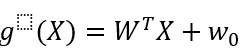其中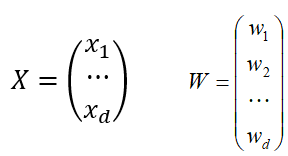Fisher选择投影方向W的原则，即使原样本向量在该方向上的投影能兼顾类间分布尽可能分开，类内样本投影尽可能密集的要求。 如下为具体步骤：

（1）W的确定

各类样本均值向量mi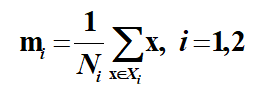样本类内离散度矩阵 和总类内离散度矩阵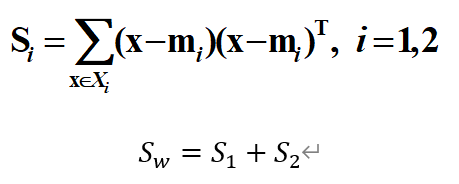样本类间离散度矩阵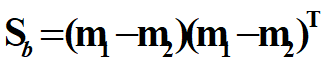在投影后的一维空间中，各类样本均值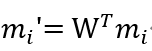样本类内离散度和总类内离散度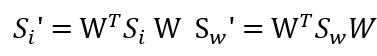样本类间离散度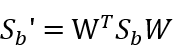Fisher准则函数为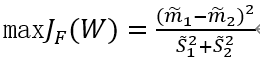（2）阈值的确定

是个常数，称为阈值权,对于两类问题的线性分类器可以采用下属决策规则:
令 则：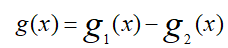如果g(x)>0,则决策x属于w1 ；如果g(x)<0,则x属于w2 ；如果g(x)=0,则可将x任意分到某一类，或拒绝。

（3）Fisher线性判别的决策规则

Fisher准则函数满足两个性质：
1.投影后，各类样本内部尽可能密集，即总类内离散度越小越好。
2.投影后，各类样本尽可能离得远，即样本类间离散度越大越好。
根据这个性质确定准则函数，根据使准则函数取得最大值，可求出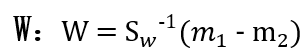这就是Fisher判别准则下的最优投影方向。
最后得到决策规则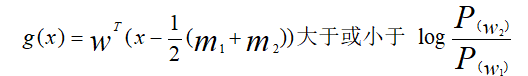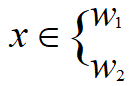对于某一个未知类别的样本向量x，如果y=WT·x>y0，则x∈w1；否则x∈w2。

二．数据描述

1.iris数据

IRIS数据集以鸢尾花的特征作为数据来源，数据集包含150个数据集，有4维，分为3 类，每类50个数据，每个数据包含4个属性，是在数据挖掘、数据分类中非常常用的测试集、训练集。

2.sonar数据

Sonar数据集包含208个数据集，有60维，分为2类，第一类为98个数据，第二类为110个数据，每个数据包含60个属性，是在数据挖掘、数据分类中非常常用的测试集、训练集。

“群内离散度”要求的是距离越远越好；而“群间离散度”的距离越近越好

三.鸢尾花数据集例子

import pandas as pd
import numpy as np
import matplotlib.pyplot as plt
import seaborn as sns
path=r'Iris.csv'
Iris1=df.values[0:50,0:4]
Iris2=df.values[50:100,0:4]
Iris3=df.values[100:150,0:4]
m1=np.mean(Iris1,axis=0)
m2=np.mean(Iris2,axis=0)
m3=np.mean(Iris3,axis=0)
s1=np.zeros((4,4))
s2=np.zeros((4,4))
s3=np.zeros((4,4))
for i in range(0,30,1):
a=Iris1[i,:]-m1
a=np.array([a])
b=a.T
s1=s1+np.dot(b,a)
for i in range(0,30,1):
c=Iris2[i,:]-m2
c=np.array([c])
d=c.T
s2=s2+np.dot(d,c)
#s2=s2+np.dot((Iris2[i,:]-m2).T,(Iris2[i,:]-m2))
for i in range(0,30,1):
a=Iris3[i,:]-m3
a=np.array([a])
b=a.T
s3=s3+np.dot(b,a)
sw12=s1+s2
sw13=s1+s3
sw23=s2+s3
#投影方向
a=np.array([m1-m2])
sw12=np.array(sw12,dtype='float')
sw13=np.array(sw13,dtype='float')
sw23=np.array(sw23,dtype='float')
#判别函数以及T
#需要先将m1-m2转化成矩阵才能进行求其转置矩阵
a=m1-m2
a=np.array([a])
a=a.T
b=m1-m3
b=np.array([b])
b=b.T
c=m2-m3
c=np.array([c])
c=c.T
w12=(np.dot(np.linalg.inv(sw12),a)).T
w13=(np.dot(np.linalg.inv(sw13),b)).T
w23=(np.dot(np.linalg.inv(sw23),c)).T
#print(m1+m2) #1x4维度  invsw12 4x4维度  m1-m2 4x1维度
T12=-0.5*(np.dot(np.dot((m1+m2),np.linalg.inv(sw12)),a))
T13=-0.5*(np.dot(np.dot((m1+m3),np.linalg.inv(sw13)),b))
T23=-0.5*(np.dot(np.dot((m2+m3),np.linalg.inv(sw23)),c))
kind1=0
kind2=0
kind3=0
newiris1=[]
newiris2=[]
newiris3=[]
for i in range(30,49):
x=Iris1[i,:]
x=np.array([x])
g12=np.dot(w12,x.T)+T12
g13=np.dot(w13,x.T)+T13
g23=np.dot(w23,x.T)+T23
if g12>0 and g13>0:
newiris1.extend(x)
kind1=kind1+1
elif g12<0 and g23>0:
newiris2.extend(x)
elif g13<0 and g23<0 :
newiris3.extend(x)
#print(newiris1)
for i in range(30,49):
x=Iris2[i,:]
x=np.array([x])
g12=np.dot(w12,x.T)+T12
g13=np.dot(w13,x.T)+T13
g23=np.dot(w23,x.T)+T23
if g12>0 and g13>0:
newiris1.extend(x)
elif g12<0 and g23>0:

newiris2.extend(x)
kind2=kind2+1
elif g13<0 and g23<0 :
newiris3.extend(x)
for i in range(30,50):
x=Iris3[i,:]
x=np.array([x])
g12=np.dot(w12,x.T)+T12
g13=np.dot(w13,x.T)+T13
g23=np.dot(w23,x.T)+T23
if g12>0 and g13>0:
newiris1.extend(x)
elif g12<0 and g23>0:
newiris2.extend(x)
elif g13<0 and g23<0 :
newiris3.extend(x)
kind3=kind3+1
correct=(kind1+kind2+kind3)/60
print("样本类内离散度矩阵S1：",s1,'\n')
print("样本类内离散度矩阵S2：",s2,'\n')
print("样本类内离散度矩阵S3：",s3,'\n')
print('-----------------------------------------------------------------------------------------------')
print("总体类内离散度矩阵Sw12：",sw12,'\n')
print("总体类内离散度矩阵Sw13：",sw13,'\n')
print("总体类内离散度矩阵Sw23：",sw23,'\n')
print('-----------------------------------------------------------------------------------------------')
print('判断出来的综合正确率：',correct*100,'%')

四.python代码推导

1.数据生成

scikit-learn的接口来生成数据：

from sklearn.datasets import make_multilabel_classification
import numpy as np

x, y = make_multilabel_classification(n_samples=20, n_features=2,
n_labels=1, n_classes=1,
random_state=2)  # 设置随机数种子，保证每次产生相同的数据。

# 根据类别分个类
index1 = np.array([index for (index, value) in enumerate(y) if value == 0])  # 获取类别1的indexs
index2 = np.array([index for (index, value) in enumerate(y) if value == 1])  # 获取类别2的indexs

c_1 = x[index1]   # 类别1的所有数据(x1, x2) in X_1
c_2 = x[index2]  # 类别2的所有数据(x1, x2) in X_2

2.fisher算法实现

def cal_cov_and_avg(samples):
u1 = np.mean(samples, axis=0)
cov_m = np.zeros((samples.shape, samples.shape))
for s in samples:
t = s - u1
cov_m += t * t.reshape(2, 1)
return cov_m, u1
def fisher(c_1, c_2):
cov_1, u1 = cal_cov_and_avg(c_1)
cov_2, u2 = cal_cov_and_avg(c_2)
s_w = cov_1 + cov_2
u, s, v = np.linalg.svd(s_w)  # 奇异值分解
s_w_inv = np.dot(np.dot(v.T, np.linalg.inv(np.diag(s))), u.T)
return np.dot(s_w_inv, u1 - u2)

3.判定类别

def judge(sample, w, c_1, c_2):
u1 = np.mean(c_1, axis=0)
u2 = np.mean(c_2, axis=0)
center_1 = np.dot(w.T, u1)
center_2 = np.dot(w.T, u2)
pos = np.dot(w.T, sample)
return abs(pos - center_1) < abs(pos - center_2)
w = fisher(c_1, c_2)  # 调用函数，得到参数w
out = judge(c_1, w, c_1, c_2)   # 判断所属的类别
print(out)

4.绘图

在jupyter下面绘制需要添加以下代码：

%matplotlib inline

这样才能在当前的jupyter下显示出图片

import matplotlib.pyplot as plt
%matplotlib inline
plt.scatter(c_1[:, 0], c_1[:, 1], c='#99CC99')
plt.scatter(c_2[:, 0], c_2[:, 1], c='#FFCC00')
line_x = np.arange(min(np.min(c_1[:, 0]), np.min(c_2[:, 0])),
max(np.max(c_1[:, 0]), np.max(c_2[:, 0])),
step=1)

line_y = - (w * line_x) / w
plt.plot(line_x, line_y)
plt.show()

运行结果：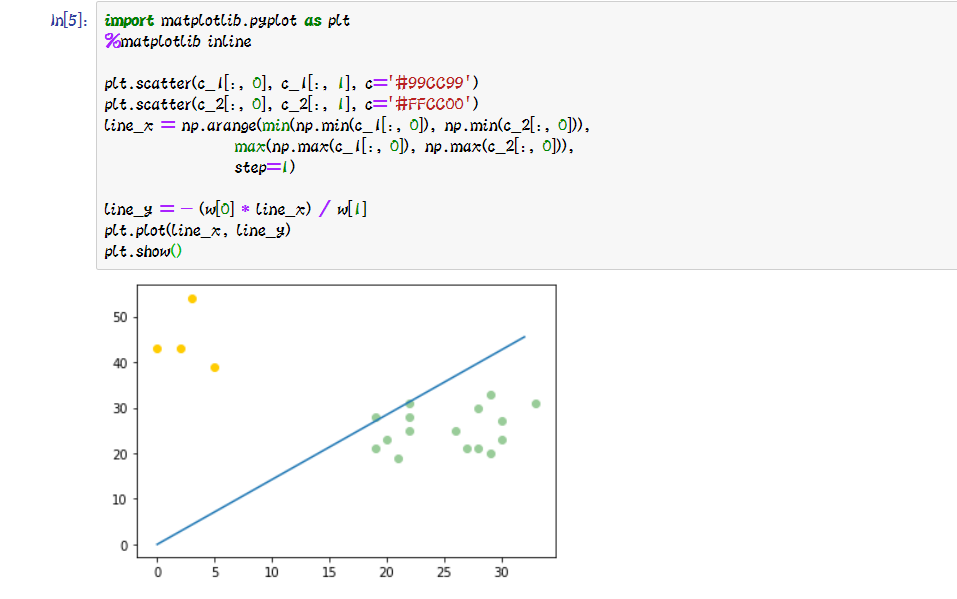五.理解和心得

Fisher判别法是判别分析的方法之一。Fisher判别法是一种投影方法，把高维空间的点向低维空间投影。在原来的坐标系下，可能很难把样品分开，而投影后可能区别明显。一般说，可以先投影到一维空间(直线)上，如果效果不理想，在投影到另一条直线上(从而构成二维空间)，依此类推。每个投影可以建立一个判别函数。

六.参考链接

展开全文机器学习 python 算法 深度学习
• 目录线性分类的数学基础python代码完成Fisher判别的推导Iris数据集数据可视化relplotjointplotdistplotboxplotviolinplotpairplot构建模型 线性分类的数学基础 1.假设对一模式记抽取n个特征,表示为: X=(x1,x2,x3,…,...
• 线性判别分析是常用的降维技术,在模式分类和机器学习的预处理步骤中。其目标是将数据集投影到具有良好的类可分性的低维空间中，以避免过度拟合（维数过多）并降低计算成本，如将一个特征空间(一个数据集n维样本)投射...

1、线性判别原理

线性判别分析是常用的降维技术,在模式分类和机器学习的预处理步骤中。其目标是将数据集投影到具有良好的类可分性的低维空间中，以避免过度拟合（维数过多）并降低计算成本，如将一个特征空间(一个数据集n维样本)投射到一个更小的子空间k(其中k ≤n-1)上，同时维护类区分信息。

判别式是一个函数，它接受一个输入向量x，并把它赋值给K个类中的一个，记作。在这一章中，我们将把注意力限制在线性判别式上，即那些决策面是超平面的判别式。为了简化讨论，我们首先考虑两个类的情况，然后研究K > 2类的扩展。

1.1 二分类

假设判别面的判别式为： （因为是线性所以可以直接这么写）

,则向量x属于类别；若,则向量x属于类别。所以决策边界由决定。

假设有两个点  和  都在决策面上，则 ,所以向量 w与决策面上的向量正交且w决定了决策面的方向。

例：假设有三个点分别在判别面上方，判别面上，判别面下方。则：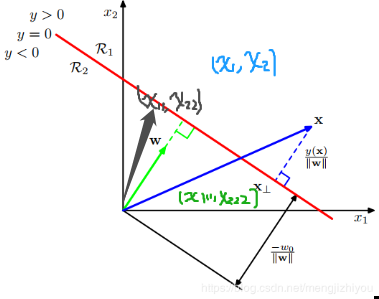,  则,因此决策边界的方程式也可以看作原数据点到决策面的距离。

原点到决策面的距离为(用的决策面上的点)：

若向量x是决策面外的点，则点到面的距离

如上图所示，向量x在决策面y=0上的投影点为向量,与决策面正交，所以与权重向量w平行，因此向量x可以表示为：

因为 ，所以：

简化，便于后面计算：

注：这里的向量默认的都是列向量,单位法向量是长度为1的法向量=向量/向量的模

1.2 多分类

现在考虑k>2类的情况：

•  一对多（one-versus-the-rest），复杂度K，会导致模糊区域，即数据不属于任何类别
• 一对一（one-versus-one），复杂度K(K - 1)/2，也会导致模糊区域

可以通过考虑一个包含K个线性函数的K类判别器来避免这些问题，则K个类别的判别式形式可以写成：

如果,则点x属于类别。因此类别和类别的决策面是，所以对于超平面可以定义为：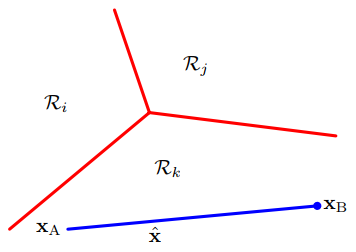上图中，均属于类别，则两点连线上的任意点可以表示为：

对于线性判别式，则有：

2 、Fisher线性判别分析方法

两个类别：

通过调整权重向量w，找到类分离的最大投影。假设类别个点，类别个点，则两个类别的平均向量为：

度量类分离最简单的方法是投影类到w上(看下图理解），说明可以选择w来最大化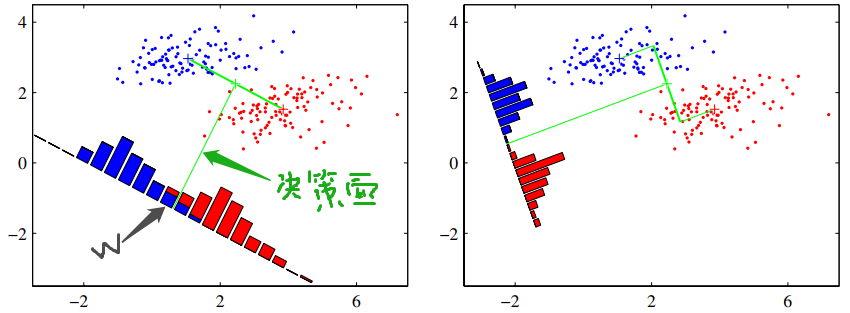左边的图显示了来自两个类的样本(用红色和蓝色表示)，以及在连接类的直线上投影得到的直方图。请注意，在投影空间中有相当多的类重叠。右边的图显示了基于Fisher线性判别式的对应投影，显示了极大地改进了类分离。

类别投影数据的均值可以表示为：， 这个表达式可以通过简单地增加w的大小而变得任意大，为此可以让向量w的长度为1，则有。通过引入拉格朗日乘子(拉格朗日乘子)来控制最大化，发现。

根据上面两个式子以及拉格朗日乘子得到：

然而，这种方法仍然存在一个问题，如上图所示。这显示了两个类在原始的二维空间(x1, x2)中被很好地分离，但是当它们被投影到连接它们的平均值的直线上时，它们有相当大的重叠。

这种困难来自于类分布的强非对角协方差。Fisher提出的思想是最大化一个函数，该函数将在预计的类均值之间提供一个大的分离，同时在每个类中提供一个小的方差，从而最小化类重叠。

类别转换后的数据即投影后的数据y的类内方差是：

整个数据集的类内协方差可以定义为：, Fisher准则定义类间协方差与类内协方差的比值：

根据与w的关系，可以写为：

对 w求导，得：

lambda 是特征值，w是特征向量。另一种理解方式：

=1

根据拉格朗日乘子：，其它同上。

与同向，此外，因为不考虑w的长度（分子分母均是w的二次项，因此解与w的长度无关），只考虑其方向，所以可以去除伸缩因子,然后两边同乘，则有：

多个类别：

考虑 K>2 的类别，假设数据维度D要远大于K。的计算同上。

为了找到  的一般形式，为此引入总协方差矩阵(total covariance matrix):

m:所有数据集的平均值，每个类别的数量，N数据集的总数量,，K是总类别数量，k指某个类别3、Python3实现

先观察每个特征的分布情况，LDA假设数据是正态分布的，特征是统计独立的，并且每个类都有相同的协方差矩阵。但是，这只适用于LDA作为分类器，如果违背了这些假设，那么降维的LDA也可以很好地工作。

from sklearn import datasets
import matplotlib.pyplot as plt
import numpy as np
import math

# prepare the data
X = iris.data
y = iris.target
names = iris.feature_names
labels = iris.target_names
y_c = np.unique(y)

"""visualize the distributions of the four different features in 1-dimensional histograms"""
fig, axes = plt.subplots(2, 2, figsize=(12, 6))
for ax, column in zip(axes.ravel(), range(X.shape)):
# set bin sizes
min_b = math.floor(np.min(X[:, column]))
max_b = math.ceil(np.max(X[:, column]))
bins = np.linspace(min_b, max_b, 25)

# plotting the histograms
for i, color in zip(y_c, ('blue', 'red', 'green')):
ax.hist(X[y == i, column], color=color, label='class %s' % labels[i],
bins=bins, alpha=0.5, )
ylims = ax.get_ylim()

# plot annotation
l = ax.legend(loc='upper right', fancybox=True, fontsize=8)
l.get_frame().set_alpha(0.5)
ax.set_ylim([0, max(ylims) + 2])
ax.set_xlabel(names[column])
ax.set_title('Iris histogram feature %s' % str(column + 1))

# hide axis ticks
ax.tick_params(axis='both', which='both', bottom=False, top=False, left=False, right=False,
labelbottom=True, labelleft=True)

# remove axis spines
ax.spines['top'].set_visible(False)
ax.spines["right"].set_visible(False)
ax.spines["bottom"].set_visible(False)
ax.spines["left"].set_visible(False)

axes.set_ylabel('count')
axes.set_ylabel('count')
fig.tight_layout()
plt.show()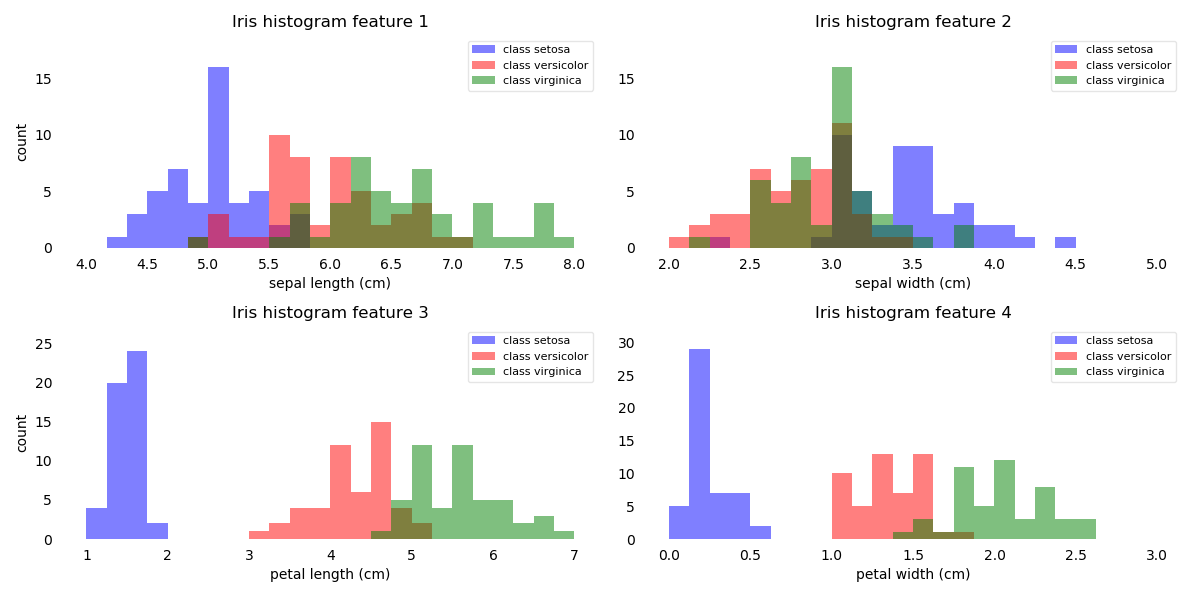这些简单的特征图形表示，花瓣的长度和宽度可能更适合分类。

Step 1: Computing the d-dimensional mean vectors

np.set_printoptions(precision=4)

mean_vector = []  # 类别的平均值
for i in y_c:
mean_vector.append(np.mean(X[y == i], axis=0))
print('Mean Vector class %s:%s\n' % (i, mean_vector[i]))

Step 2: Computing the Scatter Matrices

"""within-class scatter matrix"""
S_W = np.zeros((X.shape, X.shape))
for i in y_c:
Xi = X[y == i] - mean_vector[i]
S_W += np.mat(Xi).T * np.mat(Xi)
print('within-class scatter matrix:\n', S_W)

"""between-class scatter matrix """
S_B = np.zeros((X.shape, X.shape))
mu = np.mean(X, axis=0)  # 所有样本平均值
for i in y_c:
Ni = len(X[y == i])
S_B += Ni * np.mat(mean_vector[i] - mu).T * np.mat(mean_vector[i] - mu)
print('within-class scatter matrix:\n', S_B)

Step 3: Solving the generalized eigenvalue problem for the matrix

eigvals, eigvecs = np.linalg.eig(np.linalg.inv(S_W) * S_B)  # 求特征值，特征向量
np.testing.assert_array_almost_equal(np.mat(np.linalg.inv(S_W) * S_B) * np.mat(eigvecs[:, 0].reshape(4, 1)),
eigvals * np.mat(eigvecs[:, 0].reshape(4, 1)), decimal=6, err_msg='',
verbose=True)

Step 4: Selecting linear discriminants for the new feature subspace

# sorting the eigenvectors by decreasing eigenvalues
eig_pairs = [(np.abs(eigvals[i]), eigvecs[:, i]) for i in range(len(eigvals))]
eig_pairs = sorted(eig_pairs, key=lambda k: k, reverse=True)
W = np.hstack((eig_pairs.reshape(4, 1), eig_pairs.reshape(4, 1)))

Step 5: Transforming the samples onto the new subspace

X_trans = X.dot(W)
assert X_trans.shape == (150, 2)

对比sklearn

plt.figure(figsize=(8, 4))
plt.subplot(121)
plt.scatter(X_trans[y == 0, 0], X_trans[y == 0, 1], c='r')
plt.scatter(X_trans[y == 1, 0], X_trans[y == 1, 1], c='g')
plt.scatter(X_trans[y == 2, 0], X_trans[y == 2, 1], c='b')
plt.title('my LDA')
plt.xlabel('LD1')
plt.ylabel('LD2')
plt.legend(labels, loc='best', fancybox=True)

from sklearn.discriminant_analysis import LinearDiscriminantAnalysis

X_trans2 = LinearDiscriminantAnalysis(n_components=2).fit_transform(X, y)
plt.subplot(122)
plt.scatter(X_trans2[y == 0, 0], X_trans2[y == 0, 1], c='r')
plt.scatter(X_trans2[y == 1, 0], X_trans2[y == 1, 1], c='g')
plt.scatter(X_trans2[y == 2, 0], X_trans2[y == 2, 1], c='b')
plt.title('sklearn LDA')
plt.xlabel('LD1')
plt.ylabel('LD2')
plt.legend(labels, loc='best', fancybox=True)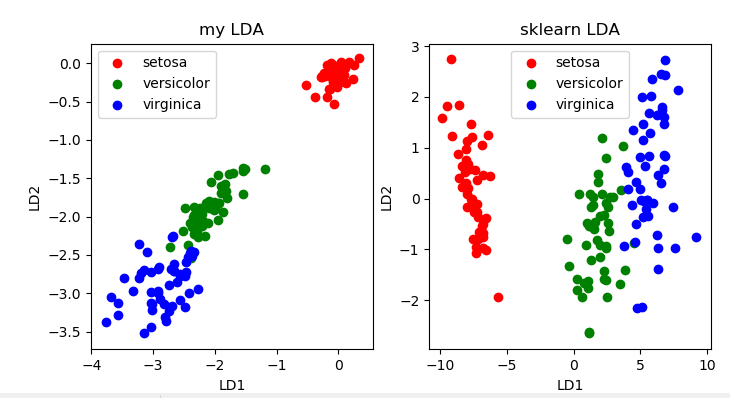展开全文• python代码完成Fisher判别的推导一、Fisher算法的主要思想二、Fisher数学算法步骤①计算各类样本均值向量mim_imi​,mim_imi​是各个类的均值，NiN_iNi​是wiw_iwi​类的样本个数。②计算样本类内离散度矩阵SiS_iSi​...
• LDA 两类Fisher线性判别分析及python实现

万次阅读 热门讨论 2016-03-19 23:23:27
参考：《模式识别》(第三版)第4.3章－Fisher线性判别分析 机器学习中的数学(4)－线性判别分析(LDA)，主成分分析(PCA)：http://www.cnblogs.com/LeftNotEasy/archive/2011/01/08/lda-and-pca-machine-learning.html ...机器学习 模式识别 python LDA
• 内容简介线性判别分析LDA的基本概念代码实例：第一部分使用python详细说明了LDA的计算过程； 第二部分记录了如何使用sklearn完成LDA。什么是线性判别分析？LDA，全名 Linear Discrimination Analysis， 是一种由监督...
• 一、Fisher线性判别分析原理解析与算法描述 Fisher：1890-1962, 英国数学家，生物学家，现代统计学奠基人之一，证明了孟德尔的遗传律符合达尔文的进化论。 Fisher线性判别分析(Linear Discriminant Analysis)是一种...机器学习
• Fisher判别分析，或称为线性判别分析（Linear Discriminant Analysis，LDA）。LDA有时也被称为Fisher’s LDA。最初于1936年，提出Fisher线性判别，后来于1948年，进行改进成如今所说的LDA。 关于Fisher判别的数学...python 机器学习
• Fisher判别分析

千次阅读 2018-02-26 09:21:57
Fisher判别分析首先我们得搞清楚什么是Fisher算法？选取任何一本模式识别与智能计算的书都有这方面的讲解。首先得知道Fisher线性判别函数，在处理数据的时候，我们经常遇到高维数据，这个时候往往就会遇到“维数灾难...
• 文章目录线性判别函数基本概念Fisher线性判别分析基本思想最优方向推导过程转换为判别函数完整代码 线性判别函数基本概念 我们主要讨论在两类情况下判别函数为线性的情况，这里给出一般情况：g(x)=wTxg(\bf{x})=\bf{...python 算法 模式识别
• 目录python代码实现Fisher判别推导一、Fisher判别法1、Fisher判别法基本定义二、Fisher判别法求解步骤1、计算各类样本均值向量2、计算样本类内离散度矩阵SiS_iSi​和总类内离散度矩阵SωS_\omegaSω​3、计算样本类...python 人工智能
• 贝叶斯判别规则是把某特征矢量(x) 落入某类集群的条件概率当成分类判别函数(概率判别函数)，x落入某集群的条件概率最大的类为X的类别，这种判决规则就是贝叶斯判别规则。贝叶斯判别规则是以错分概率或风险最小为准则...机器学习 分类
• 为了进一步理解和掌握Fisher线性判别法的基本原理和实现过程，利用Fisher判别法解决实际问题进行试验。 实验环境 Windows 10，Python 3.7.4，PyCharm 2019.2.3 实验原理 Fisher线性判别法的基本思想是：通过...Fisher Python
• 机器学习之Fisher判别分析

千次阅读 2020-05-05 18:57:58
机器学习之Fisher判别分析一、算法描述1、W的确定2、阈值的确定3、Fisher线性判别的决策规则4、群内离散度”（样本类内离散矩阵）、“群间离散度”（总类内离散矩阵）二、Python代码实现 一、算法描述 Fisher线性...python 机器学习 人工智能
• 判别分析的原理及代码 import numpy as np from sklearn.neighbors import KNeighborsClassifier X0=np.array([[1.24,1.27],[1.36,1.74],[1.38,1.90],[1.38,1.82],[1.38,1.90],[1.40,1.70],[1.48,1.82],[1.54,...
• 在理解Fisher线性分类的参考代码基础上（matlab代码），改用python代码完成Fisher判别的推导。重点理解“群内离散度”（样本类内离散矩阵）、“群间离散度”（总类内离散矩阵）的概念和几何意义。 一、Fisher线性...python
• 上一篇我们介绍了Fisher线性判别分析的原理及实现，而在判别分析中还有一个很重要的分支叫做二次判别，本文就对二次判别进行介绍：二次判别属于距离判别法中的内容，以两总体距离判别法为例，对总体G1,，G2，当他们...python判别分析
• 基于半监督局部Fisher判别分析的高光谱图像稀疏度降低研究论文
• 线性判别分析（linear discriminant analysis），LDA。也称为Fisher线性判别（FLD）是模式识别的经典算法。 （1）中心思想：将高维的样本投影到最佳鉴别矢量空间，来达到抽取分类信息和压缩特种空间维数的效果，投影...
• 之前数篇博客我们比较了几种具有...而本文便要介绍分类算法中比较古老的线性判别分析：线性判别最早提出合理的判别分析法者是R.A.Fisher(1936)，Fisher提出将线性判别函数用于花卉分类上，将花卉的各种特征利用线性...
• 在这里我们主要讨论fisher判别分析的方法。 fishter原理 费歇（FISHER判别思想是投影，使多维问题简化为一维问题来处理。选择一个适当的投影轴,使所有的样品点都投影到这个轴上得到一个投影值。对这个投影轴的...人工智能 机器学习 python
• Fisher线性判别Fisher判别法介绍Fisher线性判别Fisher准则函数的定义python代码实现 Fisher判别法介绍 Fisher判别法是判别分析的方法之一，它是借助于方差分析的思想，利用已知各总体抽取的样品的p维观察值构造一个......

fisher判别分析pythonpython 订阅